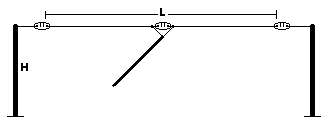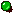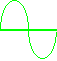e Ham Radio ~ Ham Tools

 Dipole AntennaAntenna   CalculatorEnter Results ^ Enter value then click a function key - fo=freq. (MHz)    L=1/2 wave ant. (feet) Copyright © Charlie, W5AM (Radioing.com).

 ~ Calculator Notes ~ fo = Antenna tuned frequency in MHz. L = Length (feet) of a 1/2 wave ant. (dipole), based on 95% of electrical wavelength, considering end effects. f(MHz) = Signal frequency in MHz. M = Signal wavelength (l) in meters. VSWR = Voltage Standing Wave Ratio (n). Pr = Reflected Power (%), expressed as a percentage of the incident power for a specific VSWR. P = Power (watts) into antenna feedpoint. Applies to antenna far-field calculations using E(V/m). E(V/m) = Electric field strength in volts per meter (V/m) at a distance (d = 1 meter) from an antenna with unity gain (G=1).  For other values of d, divide the calculated E(V/m) by 10 for d = 10 m; by 100 for d = 100 m, by 1000 for d = 1000 m, by 10000 for d = 10000 m, etc.  Note: This function applies to far-field calculations only (d > l/2p).  Far-field is generally at least 1/6 wavelengths from the antenna.  Based on E(V/m) = (5.5*sqrt(PG))/d. m = Meters, unit of length (39.37 inches). W/m2 = Watts per square meter. mW/cm2 = Milliwatts per square centimeter. V/m = Volts per meter. ~ Antenna Notes ~ 1/2 Wavelength Dipole: Length L(feet) = 468/f(MHz). Length L(meters) = 142.5/f(MHz).  Add 4.7 percent to L for an Inverted V configuration, depending on apex angle. The antenna height (H) above terrain should be 20 feet minimum.  Each leg should be 1/2 of the calculated ant. length (L), with several inches added for tuning and for securing to insulators.  Actual resonant antenna lengths can vary with antenna height, ground conductivity, and proximity of surrounding objects.  Start with a longer antenna length and adjust the legs in equal increments for minimum VSWR.  Antenna feedline choice is optional, but you should consider the output matching capability of the transmitter.  Coaxial cable is preferred unless the antenna is for multi-band operation.  A 50 Ohm coaxial feedline is recommended for Inverted V antennas.  It should also be used for dipoles at lower heights.  The antenna feed-point impedance for dipoles approaching 1/2 wavelength in height can rise to near 72 Ohms, depending on installation.

USA HF Band Limits and Modes
Band
(Meters)
Frequency (MHz)
CW, RTTY, Data
Frequency (MHz)
Voice
160 1.800 - 2.000 1.800 - 2.000
80, 75 3.500 - 3.750 3.750 - 4.000
40 7.000 - 7.150 7.150 - 7.300
30 10.100 - 10.150 None
20 14.000 - 14.150 14.150 - 14.350
17 18.068 - 18.110 18.110 - 18.168
15 21.000 - 21.200 21.200 - 21.450
12 24.890 - 24.930 24.930 - 24.990
10 28.000 - 28.300 28.300 - 29.700